# Concept: Geometry - Basics

CONTENTS

Fundamentals of Geometry

Point: It is an exact location. It is a fine dot which has neither length nor breadth nor thickness but has position i.e. it has no magnitude.

Line: A line is a set of all the points in one dimension. It can be extended in both the directions; hence, its length is infinite. A line does not have either width or thickness.

Intersecting lines: Two lines having a common point are called intersecting lines. The common point is known as the point of intersection.

Line Segment: The straight path joining two points A and B is called a line segment AB. It has end points and a definite length.

Ray: A line segment which can be extended in only one direction is called a ray.

Concurrent lines: If two or more lines intersect at the same point, then they are known as concurrent lines.

Plane: A plane is set of all the points in two dimensions. It does not have any thickness but is indefinitely extended in all directions.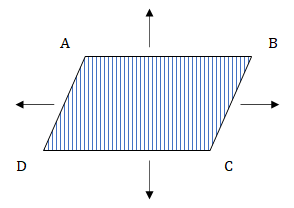​​​​​​​

Angles: when two straight lines meet at a point they form an angle.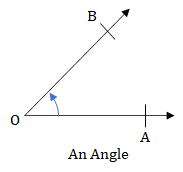​​​​​​​

In the figure above, the angle is represented as ∠AOB. OA and OB are the arms of ∠AOB. Point O is the vertex of ∠AOB. The amount of turning from one arm (OA) to other (OB) is called the measure of the angle (AOB).

Right angle: An angle whose measure is 90° is called a right angle.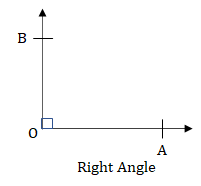​​​​​​​

Acute angle: An angle whose measure is less than one right angle (i.e. less than 90°), is called an acute angle.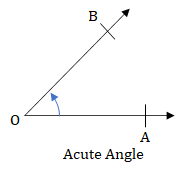​​​​​​​

Obtuse angle: An angle whose measure is more than one right angle and less than two right angles (i.e. less than 180° and more than 90°) is called an obtuse angle.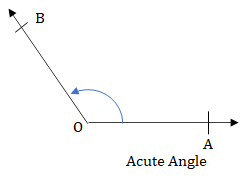​​​​​​​

Straight angle: An angle that measure exactly 180° is called a straight angle. The angle shown in the figure below is a straight angle.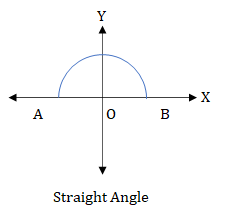​​​​​​​

Reflex angles: An angle whose measure is more than 180° and less than 360° is called a reflex angle.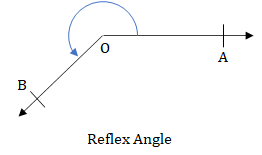​​​​​​​

Complementary angles: If the sum of the two angles is one right angle (i.e. 90°), they are called complementary angles. Therefore, the complement of an angle θ is equal to 90° - θ.​​​​​​​

Supplementary angles: Two angles are said to be supplementary, if the sum of their measures is 180°. Therefore, supplement of an angle θ is 180° - θ.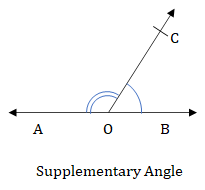​​​​​​​

Bisector of an angle: If a ray or a straight line passing through the vertex of that angle, divides the angle into two angles of equal measurement, then that line is known as the bisector of that angle.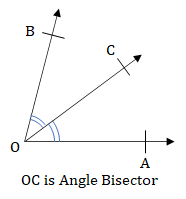​​​​​​​

A point on an angle bisector is equidistant from both the arms.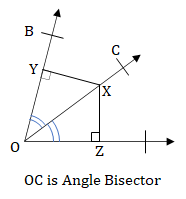​​​​​​​

In the figure above, Q and R are the feet of perpendiculars drawn from P to OB and OA. It follows that PQ = PR.

Vertically opposite angles: When two straight lines intersect each other at a point, the pairs of opposite angles so formed are called vertically opposite angles.

In the above figure, ∠1 and ∠3, ∠2 and ∠4 are vertically oppostie angles.

Vertically opposite angles are always equal.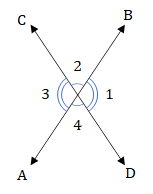​​​​​​​

Adjacent angle: When two angles share a common side and a common vertex, we get two adjacent angles. For two angles to be adjacent, no angles should be inside the other.​​​​​​​

If the sum of two adjacent angles is 180° then these angles form a linear pair.

Angles making a linear pair are supplementary to each other.

Perpendicular lines: Two lines intersecting each other at 90° are said to be perpendicular to each other.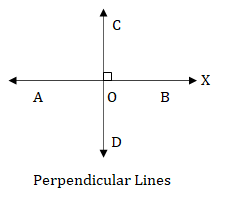​​​​​​​

Parallel lines: Two lines are parallel if they are coplanar and they do not intersect each other even if they are extended on either side.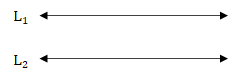​​​​​​​

Two lines in the same plane that are perpendicular to a given line are parallel to each other.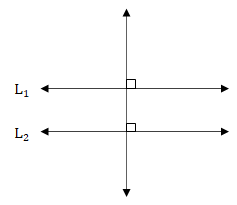​​​​​​​

Line segment bisector: A line segment bisector which makes an angle of 90° with the given segment is known as the perpendicular bisector for the given segment.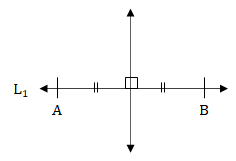​​​​​​​

Any point of the perpendicular bisector is at an equal distance from both the ends of the given line segment.​​​​​​​

Distance of a point from a line means the length of the perpendicular drawn from the point to the line. It is the shortest distance of the point from the line.​​​​​​​

Distance between two parallel lines is the perpendicular distance between them.​​​​​​​

Distance between two coplanar but non-parallel lines is always zero, because these lines intersect each other at some point.

Transversal: A transversal is a line that intersects (or cuts) two or more coplanar lines at distinct points.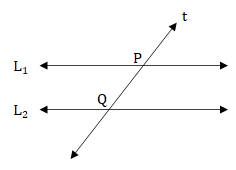​​​​​​​

In the above, figure, a transversal t is intersecting two parallel lines, l and m, at A and B, respectively.

Angles formed by a transversal of two parallel lines: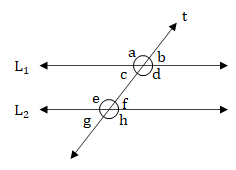​​​​​​​

In the above figure, L1 and L2 are two parallel lines intersected by a transversal. The following properties of the angles can be observed:

• Vertically opposite angles are equal: a = d, b = c, e = h and f = g
• Alternate interior angles are equal: c = f and d = e
• Alternate exterior angles are equal: a = h and b = g
• Corresponding angles are equal: a = e, b = f, c = g and d = h
• Interior angles on the same side of the transversal are supplementary: c + e = 180° and d + 180°
• Exterior angles on the same side of the transversal are supplementary: a + g = 180° and b + h = 180°

Basic Proportionality Theorem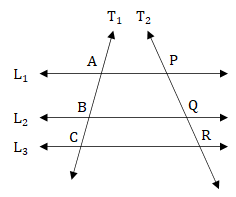​​​​​​​

For a set of three or more parallel lines (L1, L2 and L3 for example), and two transversals (T1 and T2 for example), the ratio of the lengths of the intercepts of any transversal is equal

i.e., $\frac{\mathrm{AB}}{\mathrm{BC}}$ = $\frac{\mathrm{PQ}}{\mathrm{QR}}$

## Feedback

Help us build a Free and Comprehensive CAT/MBA Preparation portal by providing us your valuable feedback about Apti4All and how it can be improved.